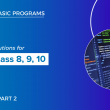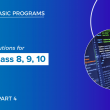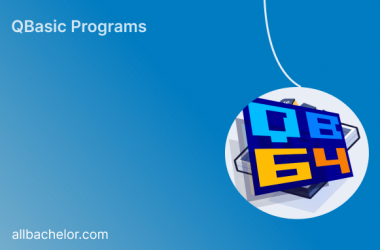# Qbasic programs solutions(part 3) for class 8, class 9 and class 10

Here, I have listed some of the qbasic programs. We also have mobile app where you can view all the qbasic programs. For better experience I highy recommend you to use our mobile app

41  Write a program to display cost of painting the four walls of a room.

``````REM PROGRAM TO COST OF PAINTING THE FOUR WALLS OF  A ROOM
CLS
INPUT “ENTER LENGTH”; L
INPUT “ENTER HEIGHT”; H
INPUT “ENTER COST OF A PAINTING”; C
A = 2 * H * (L + B)
T = C * A
PRINT “COST OF PAINTING A ROOM“; T
END
﻿``````

42  Write a program to display simple interest

``````REM PROGRAM TO DISPLAY SIMPLE INTEREST
CLS
INPUT “ENTER PRINCIPAL”; P
INPUT “ENTER TIME”; T
INPUT “ENTER RATE”;R
I = P* T * R / 100
PRINT “SIMPLE INTEREST =”; I
END ``````

43  Write a program to input number as paise and convert into rupees only.

``````CLS
INPUT "Enter paisa "; P
RS = P / 100
PRINT "Rupees value = "; RS
END ``````

44  Write a program to input selling price and cost price calculate profit or loss percentage.

``````REM PROGRAM TO CALCULATE PROFIT OR LOSS PERCENTAGE
CLS
INPUT “ENTER COST PRICE”; CP
INPUT “ENTER SELLING PRICE”; SP
IF CP > SP THEN
LP = ((CP - SP)/CP) * 100
PRINT "LOSS PERCENTAGE="; LP
ELSEIF SP > CP THEN
PP = ((SP - CP)/CP) * 100
PRINT "PROFIT PERCENTAGE"; PP
ELSE
PRINT "NEITHER PROFIT NOR LOSS"
END IF
END ``````

45  Write a program to input the day and display whether the day is Saturday or not?

``````CLS
INPUT”Enter the todays day”;d\$
IF d\$=”SATURDAY”
THEN PRINT”TODAY IS SATURDAY”
ELSE
PRINT”TODAY IS NOT SATURDAY”
END ``````

46  Write a program to ask quantity of pen    copy and pencil and their rate and find out the total amount.

``````REM CALCULATE TOTAL AMOUNT
CLS
INPUT “ENTER QUANTITY OF PEN”;QP
INPUT “ENTER RATE OF PEN”; RP
INPUT “ENTER QUANTITY OF COPY”; QC
INPUT “ENTER RATE OF COPY”; RC
INPUT “ENTER QUANTITY OF PENCIL”; QPC
INPUT” ENTER RATE OF PENCIL”; RPC
TA = (QP * RP) + (QC * RC) + (QPC * RPC)
PRINT “TOTAL AMOUNT =”; TA
END
﻿``````

47  Write a program in qbasic to convert decimal number to octal number

``````CLS
INPUT "ENTER DECIMAL NUMBER"; D
WHILE D   < >0
R = D MOD 8
S\$ = STR\$(R) + S\$
D = D \ 8
WEND
PRINT "OCTAL EQUIVALENT VALUE="; S\$
END ``````

48   Write a program in qbasic to ask number and find sum of cube of odd digits

``````REM
CLS
INPUT "ENTER ANY NUMBER"; N
S = 0
WHILE N < > 0
R = N MOD 10
IF R MOD 2 = 1 THEN S = S + R ^ 3
N = N \ 10
WEND
PRINT "SUM OF CUBE OF ODD DIGITS"; S
END
﻿``````

49  Write a program in qbasic using Sub procedure to print 1    10    101    1010    10101

``````CLS

FOR I = 1 TO 5
FOR J = 1 TO I
PRINT J MOD 2;
NEXT J
PRINT
NEXT I
END
﻿``````

50  Write a program in qbasic to find the sum of all natural numbers from 1 to 100

``````CLS
FOR I = 1 TO 100
S = S + I
NEXT I
PRINT “SUM OF ALL NATURAL NUMBERS FROM 1 TO 100=”; S
END``````

51  Write a program in qbasic to generate the following series 1   121   12321   1234321   123454321

``````CLS
A# = 1
FOR I = 1 TO 5
PRINT A# ^ 2
A# = A# * 10 + 1
NEXT I
END
﻿``````

52  Write a program in qbasic to print sum of cube of any two ask numbers

``````REM PROGRAM TO DISPLAY SUM OF CUBE
CLS
INPUT “ENTER FIRST NUMBER”; A
INPUT “ENTER SECOND NUMBER”; B
C = A ^ 3 + B ^ 3
PRINT “SUM OF CUBE OF TWO NUMBERS ”; C
END ``````

53  Write a program in qbasic to generate the series .1   0.01   0.001   0.0001   0.00001

``````CLS
A = 1
FOR I = 1 TO 5
PRINT A / 10 ^ I
NEXT I
END ``````

54  Write a program in qbasic using Sub procedure to print 50   75   100   125   150

``````CLS
CALL SERIES
FOR I = 50 TO 150 STEP 25
PRINT I,
NEXT I
END ``````

55  Write a program in qbasic to ask string and print the ask string in alternate character

``````CLS
INPUT "ENTER ANY WORD"; S\$
FOR I = 1 TO LEN(S\$)
B\$ = MID\$(S\$, I, 1)
IF I MOD 2 = 1 THEN
W\$ = W\$ + LCASE\$(B\$)
ELSE
W\$ = W\$ + UCASE\$(B\$)
END IF
NEXT I
PRINT W\$
END``````

56  Write a program in qbasic to print cube of all numbers from 1 to 50

``````    CLS
FOR I = 1 TO 50
PRINT I ^ 3,
NEXT I
END  ``````

57  Write a program in qbasic to ask three sides of a triangle and determine whether a triangle is equilateral triangle or isoceles

``````    CLS
INPUT “ENTER THREE SIDES OF A TRIANGLE”; A,B,C
IF A = B AND B = C THEN
PRINT “IT IS A EQUILATERAL TRIANGLE”
ELSE
PRINT “IT IS NOT A EQUILATERAL TRIANGLE”
END IF ``````

58  Write a program to enter any 20 numbers and display the greatest and smallest one using array

``````    CLS
DIM N(20)
FOR I = 1 TO 20
INPUT "ENTER THE NUMBERS"; N(I)
NEXT I
G = N(1)
FOR I = 2 TO 20
IF N(I) > G THEN G = N(I)
NEXT I
PRINT “THE GREATEST NUMBER IS”; G
END ``````

59  Write a program in qbasic to generate the following series 1   4   9…………9th term

``````CLS
FOR I = 1 TO 9
PRINT I ^ 2
NEXT I
END  ``````

60   Write a program in qbasic to print all Armstrong numbers from 1 to 500

``````">  CLS
INPUT "ENTER ANY NUMBER"; N
A = N
S = 0
WHILE N < > 0
R = N MOD 10
S = S + R ^ 3
N = N \ 10
WEND
IF A = S THEN
PRINT A; "IS ARMSTRONG"
ELSE
PRINT A; "IS NOT ARMSTRONG"
END IF
END ``````

##### Previous Post## Qbasic programs solutions(part 2) for class 8, class 9 and class 10

##### Next Post## Qbasic programs solutions(part 4) for class 8, class 9 and class 10

##### Related Posts## Introduction to QBASIC Syntax and Data Types: A Beginner’s Guide

What is QBASIC? QBASIC is a programming language developed by Microsoft for beginners to learn programming concepts easily.…## QBasic and BASIC

Introduction: Welcome to our journey through programming history! Today, we’ll explore two important programming languages from the past…## Looping in Qbasic

A loop is a set of instructions that is repeated until a certain result is achieved. Loops are…